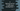# C program to find the sum of first n odd numbers starting from 1### C program to find the sum of n odd numbers starting from 1:

In this tutorial, we will learn how to find the total sum of first ’n’ odd numbers using a C program. For example , the sum of first 3 odd numbers is 1 + 3 + 5 = 9. The user will enter the value of n and our program will find out the sum and print out the answer. We will discuss two different ways to find out the sum.

### 1. Using a for or while loop :

We can find out the sum by using one for or while loop. The loop will run from 1 to n and for each number it will check if it is divisible by 2 or not. If not, means it is an odd number, so add it to a final sum variable. Finally, print out the result. The program will explain below each step how it works.

### 2. A better approach : By finding the sum of all n numbers with a difference between each numbers mathematically :

The mathematical formulae to find the sum of first n numbers starting from a with a difference d between each is :

``Sn = (n/2) [2a + (n-1)*d]``

For example, sum of first 5 numbers starting from 3 _ with a difference between each _2 is :

``````//here n = 5, a = 3 , d = 2
(5/2)[2*3 + (5-1)*2]
= (5/2)[6 + 8]
= (5/2) * 14
= 35``````

Manually , we can verify it like :

``````3 + 5 + 7 + 9 + 11
= 15 + 20
= 35``````

Our problem is to find the sum of first n odd numbers starting from 1. In this case , a = 1, d = 2 ( since the difference between two odd numbers is 2). So, if we put these values in the above formulae, it will become :

``````(n/2)[2*a + (n-1)*d]
= (n/2)[2*1 + (n-1)*2]
= (n/2)[2 + 2n - 2]
= (n/2) * 2n
= n*n
= n^2``````

That means the sum of first n odd number is square of n.

### C program explains above solutions :

In the C program below, we will explain you how to solve the problem using above two approaches. Let’a take a look :

``````#include

//4
int findUsingWhile(int n){
//5
int i = 1;
int sum = 0;
int totalOdd = 0;

//6
while(totalOdd != n){
if(i%2 != 0){
sum += i;
totalOdd++;
}
i++;
}
//7
return sum;
}

//8
int findUsingFor(int n){
//9
int i;
int sum = 0;
int totalOdd = 0;

//10
for(i=1; totalOdd != n;i++){
if(i%2 != 0){
sum += i;
totalOdd ++;
}
}
//11
return sum;
}

//12
int usingMathematics(int n){
return n*n;
}

int main(){
//1
int n;

//2
printf("Enter the value of 'n' : ");
scanf("%d",&n);

//3
printf("Total sum using while loop : %d\n",findUsingWhile(n));
printf("Total sum using for loop : %d\n",findUsingFor(n));
printf("Total sum mathematically : %d\n",usingMathematics(n));
}``````

### Explanation :

The commented numbers in the above program denote the step number below :

1. Create one integer variable n to store the value of user input number.
2. Ask the user to enter a number. Read and store it in n .
3. Calculate the sum of first n odd numbers by using one for loop, using one while loop and by using the mathematical function shown above. For each case, print out the result. All these results should be same.
4. findUsingWhile function takes the value of n as parameter and it calculates the sum using one while loop.
5. Create one variable i to use in the loop, totalOdd to total odd numbers found and sum to store the sum of all odd numbers.
6. Run one while loop. It will run continuously till total number of odd numbers becomes equal to the input n. Inside the loop, check if current value of i is odd or not. If odd, add it to the sum and increment the value of totalOdd. For each iteration, increment the value of i means we are checking it for each numbers continuously.
7. After the loop is completed, return the value of sum.
8. findUsingFor is similar to the above function. It takes the value of n as input and calculates the sum using a for loop.
9. Create three variables similarly as explained in the above function.
10. Similar to while function above, this function runs one for loop to find out the sum. It will run from i = 1 . Each time , value of i is increased by one. If the value of totalOdd becomes equal to n , this loop will end. Inside the loop, check if i is odd or even. If odd, add its value to the final sum and increment the value of totalOdd.
11. Finally, after the loop is completed, return the value of sum.
12. This is the third approach. Simply return the value of n*n. It should be the required sum as explained above.

### Sample Output :

``````Enter the value of 'n' : 9
Total sum using while loop : 81
Total sum using for loop : 81
Total sum mathematically : 81

Enter the value of 'n' : 1
Total sum using while loop : 1
Total sum using for loop : 1
Total sum mathematically : 1

Enter the value of 'n' : 3
Total sum using while loop : 9
Total sum using for loop : 9
Total sum mathematically : 9

Enter the value of 'n' : 11
Total sum using while loop : 121
Total sum using for loop : 121
Total sum mathematically : 121``````